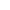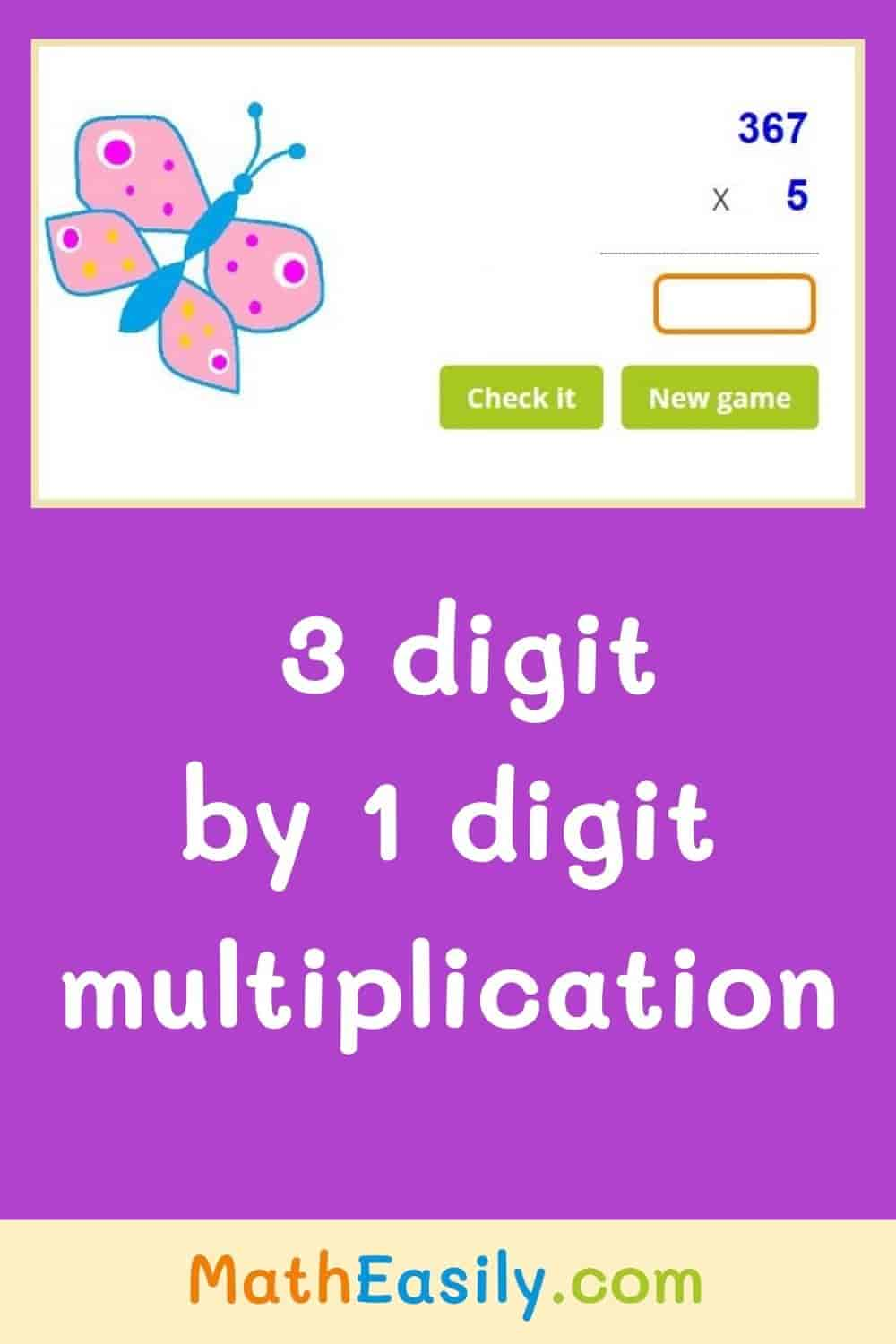﻿ 3 digit by 1 digit multiplication games and worksheets

Page No. 1089

# 3 digit by 1 digit multiplication

Multiplication 3 digit by 1 digit multiplication games online. Download three digit by one digit multiplication worksheets with answers. Enjoy our 3 by 1 digit multiplication online games. You can try also this one digit by three digit multiplication. Learn to multiply 3-digit by 1-digit numbers games for 4th graders. Play 3 digit by 1 digit multiplication online games. We prepared multiplying 3-digit by 1-digit worksheets PDF. 3 digit by 1 digit multiplication activities help kids to master 3 by 1 multiplication.

X
------------------------------------------------------------

New gameFill in the blanks with the result of 3 digit by 1 digit multiplication. Fill in only 1 digit to each empty box.

## 3 digit by 1 digit multiplication gamesInsert the result of three digit by one digit multiplication to the empty box. Press "Check it" to find out the correct answer. If your result is OK, boxes will be green.

If your answer is wrong, the wrong boxes will be red and you will see the correct answer. Press the button "New game" and play another 3 digit by 1 digit multiplication game.

This 3 digit by 1 digit multiplication game assumes that you already master single digit multiplication.

This game will help you with learning / teaching multiplication. If you are not sure how to multiply 3 digit numbers, watch this video on YouTube.

If you want to try something little bit easier then play this 2 digit by 1 digit multiplication game. Or you can try a more challenging task: 3 digit by 2 digit multiplication.

## 3 digit by 1 digit multiplication worksheets PDF (with answers)

Download our free 3 digit by 1 digit multiplication worksheets in PDF with answers, which help you to teach three digit by one digit multiplication. You can find even more free multiplication worksheets here.## 3 digit by 1 digit multiplication online games

Learn to multiply 3 digit by 1 digit numbers. Our free 3 digit by 1 digit multiplication online games are intended for kids in the 4th grade, but of course they can be played by 3rd grade math students or 5th graders.

You can play 3 digit by 1 digit multiplication games on any device, you need only internet connection.